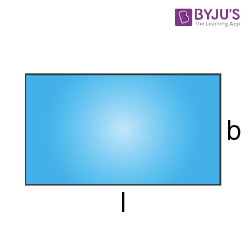Jet Set Go! All about Aeroplanes Jet Set Go! All about Aeroplanes

# Length

When we see some heights such as hills, towers, buildings, long trees, bridges, tracks, etc., we often think of how tall these are. In such cases, we tend to measure the height of these objects. This height is sometimes referred to as the length of a particular thing. For example, the length of the bridge or track and so on. In this article, you will learn the definition of length along with units, conversion rules and more information including examples in detail.

• Definition
• Units
• Conversion
• Table
• How to Measure?

## Definition

Length is defined as the measurement or extent of something from end to end. In other words, it is the larger of the two or the highest of three dimensions of geometrical shapes or objects. For example, a rectangle has its dimensions as length and breadth. Also, the length is a quantity with dimension distance in the International System of Quantities.

## Length Unit

As defined in the previous section, length is the measure of something. Now the question is how to express length in an understandable format so that everyone can understand the original aspect. The base unit for length in the International System of Units (SI) system, is the metre and it is abbreviated as m. This can be done when the length is expressed using suitable units, such as the length of a table is 2 meters or 200 cm, the length of a string is 15 meters and so on. Thus, the units of measurement will help in understanding the given parameters in numeral format.

### Length Conversion

In the metric system, length or the distance is expressed in terms of kilometers (km), meters (m), decimeter (dm), centimeters (cm), millimeters (mm). It is possible to convert units from km to m or m to cm or cm to mm and so on.

### Length Conversion Table

The conversion of units from one unit to another is essential while solving many problems to understand the parameters. Below are the few conversions which are basic and will help in problem solving.

 km to m m to cm cm to mm km m m cm cm mm 1 1000 1 100 1 10 2 2000 2 200 2 20 5 5000 10 1000 10 100 10 10000 50 5000 50 500 100 100000 100 10000 100 1000